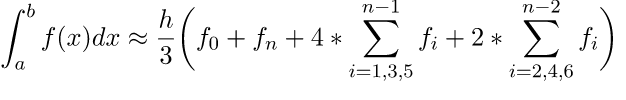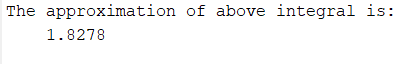GeeksforGeeks App
Open AppBrowser
Continue

## Related Articles

Simpson’s 1/3 rule is a numerical method used for the evaluation of definite integrals. MATLAB does not provide an in-built function to find numerical integration using Simpson’s rule. However, we can find that using the below formula.

The formula for numerical integration using Simpson’s rule is:`where, h = (b-a)/n`

In Simpson’s 1/3 rule, we evaluate the definite integral using integration by successive segments of the curve. It helps us to make the approximations more precise as compared to trapezoidal rule where straight lines segments were used instead of parabolic arcs.

Note: For Simpson’s 1/3 Rule, n must be even.

Example: Evaluatewithin limit 4 to 5.2

## Matlab

 `% MATLAB code for syms function that creates a variable``% dynamically and automatically assigns``% to a MATLAB variable with the same name``syms x`` ` `% Lower Limit``a = 4;`` ` `% Upper Limit``b = 5.2;`` ` `% Number of Segments``n = 6;`` ` `% Declare the function``f1 = log(x);`` ` `% inline creates a function of string containing in f1``f = inline(f1);`` ` `% h is the segment size``h = (b - a)/n;`` ` `% X stores the summation of first and last segment``X = f(a)+f(b);`` ` `% variables Odd and Even to store ``% summation of odd and even``% terms respectively``Odd = 0;``Even = 0;``for` `i = 1:2:n-1``    ``xi=a+(i*h);``    ``Odd=Odd+f(xi);``end``for` `i = 2:2:n-2``    ``xi=a+(i*h);``    ``Even=Even+f(xi);``end`` ` `% Formula to calculate numerical integration ``% using Simpsons 1/3 Rule``I = (h/3)*(X+4*Odd+2*Even);`` ` `disp(``'The approximation of above integral is: '``);``disp(I);`

Output:My Personal Notes arrow_drop_up